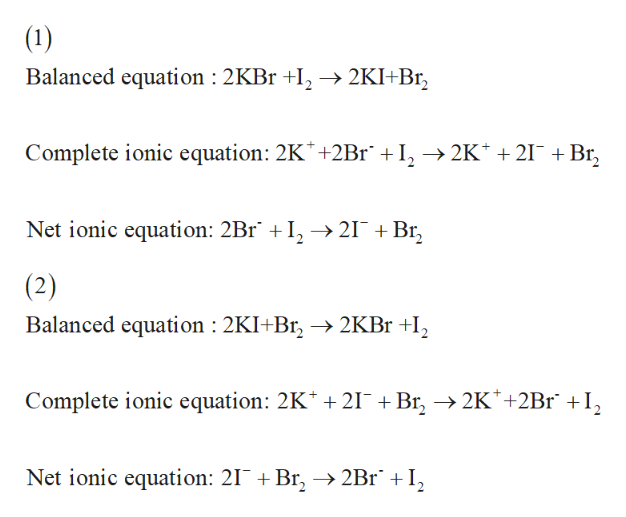# Write the Net-Ionic Reaction for the following. 1) KBr + I2 =2) KI + Br2 = 3) FeCl3 + KBr = 4) FeCl3 + KI = 5) Br2 + Cu 6) I2 + Cu =

Question
247 views

Write the Net-Ionic Reaction for the following.

1) KBr + I2 =

2) KI + Br2 =

3) FeCl3 + KBr =

4) FeCl3 + KI =

5) Br2 + Cu

6) I+ Cu =

check_circle

Step 1

“Hey, since there are multiple subparts posted, we will answer first three questions. If you want any specific question to be answered then please submit that question only or specify the question number in your message.”

Step 2help_outlineImage Transcriptionclose(1) Balanced equation : 2KBr +I2 -> 2KI+Br2 Complete ionic equation: 2K +2Br +I, >2K 21 +Br Net ionic equation: 2Br I -> 21 + Br (2) Balanced equation : 2KI+Br2KBr +I Complete ionic equation: 2K 21 Br 2K+2Br +I Net ionic equation: 21 + Br, -»2Br + I, fullscreen

### Want to see the full answer?

See Solution

#### Want to see this answer and more?

Solutions are written by subject experts who are available 24/7. Questions are typically answered within 1 hour.*

See Solution
*Response times may vary by subject and question.
Tagged in

### General Chemistry